Anúncio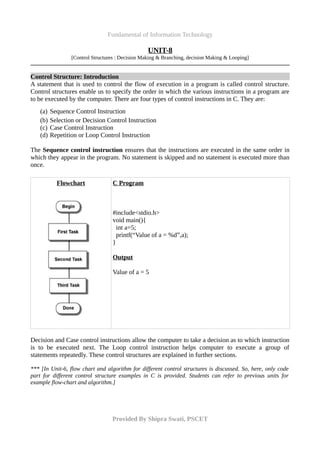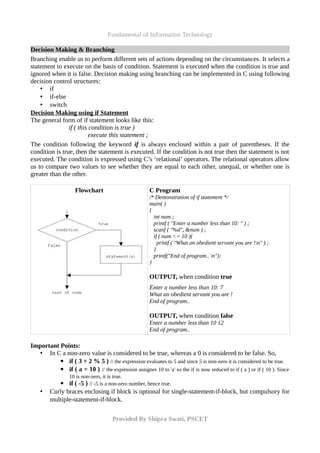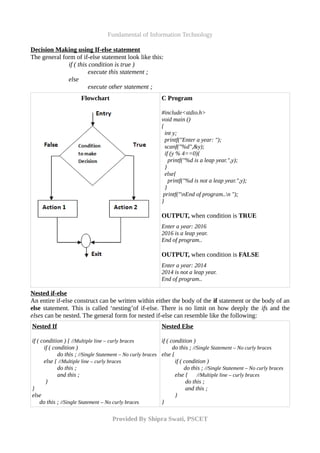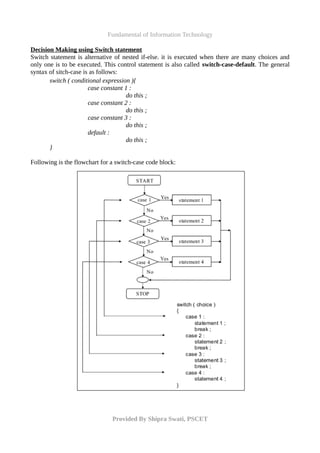Anúncio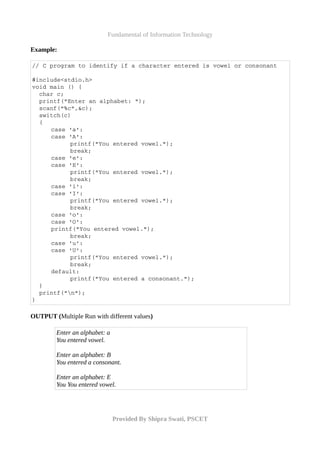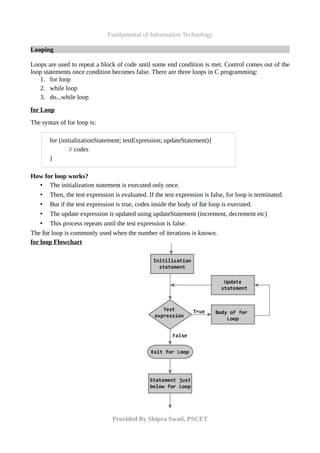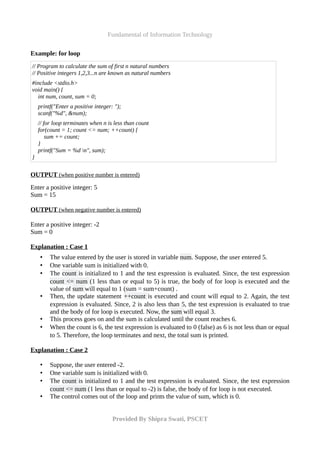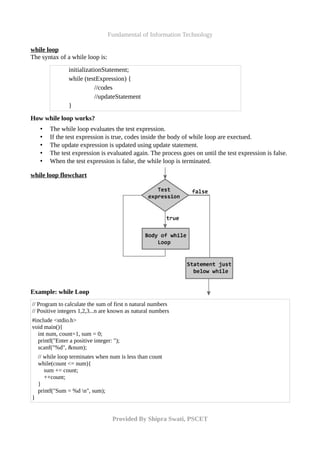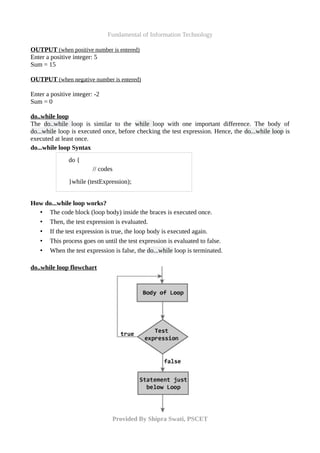Anúncio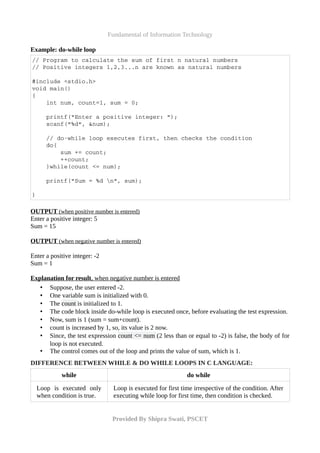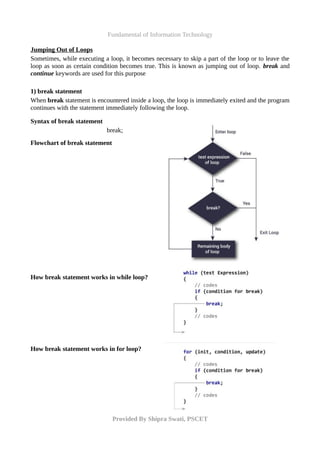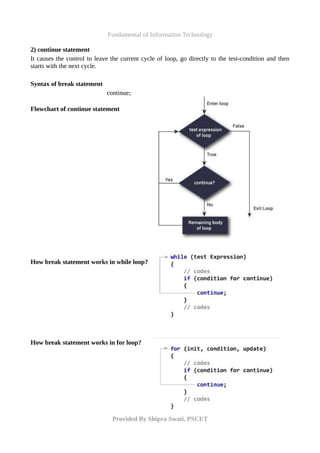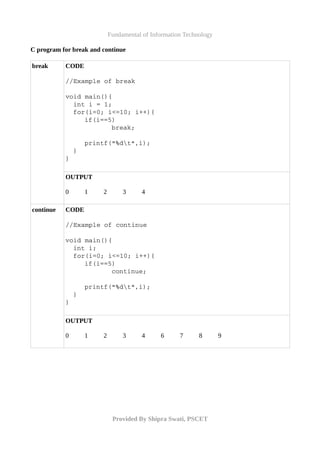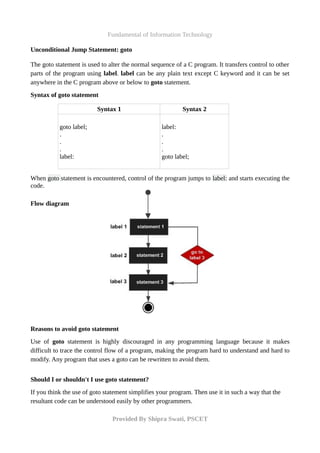Anúncio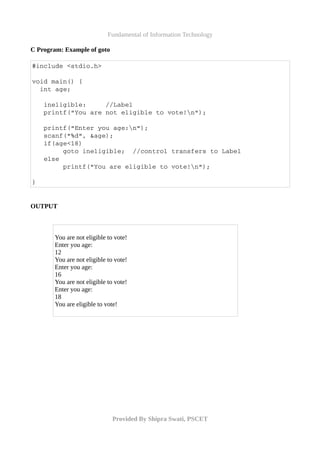Próximos SlideShares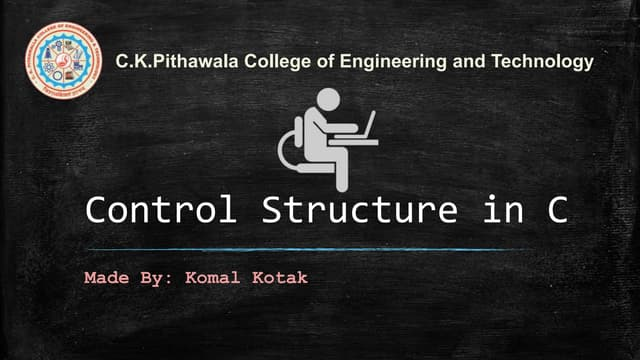Control structure of c
Carregando em ... 3
1 de 15
Anúncio

### Fundamental of Information Technology - UNIT 8

1. Fundamental of Information Technology UNIT-8 [Control Structures : Decision Making & Branching, decision Making & Looping] Control Structure: Introduction A statement that is used to control the flow of execution in a program is called control structure. Control structures enable us to specify the order in which the various instructions in a program are to be executed by the computer. There are four types of control instructions in C. They are: (a) Sequence Control Instruction (b) Selection or Decision Control Instruction (c) Case Control Instruction (d) Repetition or Loop Control Instruction The Sequence control instruction ensures that the instructions are executed in the same order in which they appear in the program. No statement is skipped and no statement is executed more than once. Flowchart C Program #include<stdio.h> void main(){ int a=5; printf(“Value of a = %d”,a); } Output Value of a = 5 Decision and Case control instructions allow the computer to take a decision as to which instruction is to be executed next. The Loop control instruction helps computer to execute a group of statements repeatedly. These control structures are explained in further sections. *** [In Unit-6, flow chart and algorithm for different control structures is discussed. So, here, only code part for different control structure examples in C is provided. Students can refer to previous units for example flow-chart and algorithm.] Provided By Shipra Swati, PSCET
2. Fundamental of Information Technology Decision Making & Branching Branching enable us to perform different sets of actions depending on the circumstances. It selects a statement to execute on the basis of condition. Statement is executed when the condition is true and ignored when it is false. Decision making using branching can be implemented in C using following decision control structures: • if • if-else • switch Decision Making using if Statement The general form of if statement looks like this: if ( this condition is true ) execute this statement ; The condition following the keyword if is always enclosed within a pair of parentheses. If the condition is true, then the statement is executed. If the condition is not true then the statement is not executed. The condition is expressed using C’s ‘relational’ operators. The relational operators allow us to compare two values to see whether they are equal to each other, unequal, or whether one is greater than the other. Flowchart C Program /* Demonstration of if statement */ main( ) { int num ; printf ( "Enter a number less than 10: " ) ; scanf ( "%d", &num ) ; if ( num <= 10 ){ printf ( "What an obedient servant you are !n" ) ; } printf("End of program.. n"); } OUTPUT, when condition true Enter a number less than 10: 7 What an obedient servant you are ! End of program.. OUTPUT, when condition false Enter a number less than 10 12 End of program.. Important Points: • In C a non-zero value is considered to be true, whereas a 0 is considered to be false. So, ▪ if ( 3 + 2 % 5 ) // the expression evaluates to 5 and since 5 is non-zero it is considered to be true. ▪ if ( a = 10 ) // the expression assignes 10 to 'a' so the if is now reduced to if ( a ) or if ( 10 ). Since 10 is non-zero, it is true. ▪ if ( -5 ) // -5 is a non-zero number, hence true. • Curly braces enclosing if block is optional for single-statement-if-block, but compulsory for multiple-statement-if-block. Provided By Shipra Swati, PSCET
3. Fundamental of Information Technology Decision Making using If-else statement The general form of if-else statement look like this: if ( this condition is true ) execute this statement ; else execute other statement ; Flowchart C Program #include<stdio.h> void main () { int y; printf("Enter a year: "); scanf("%d",&y); if (y % 4==0){ printf("%d is a leap year.",y); } else{ printf("%d is not a leap year.",y); } printf("nEnd of program..n "); } OUTPUT, when condition is TRUE Enter a year: 2016 2016 is a leap year. End of program.. OUTPUT, when condition is FALSE Enter a year: 2014 2014 is not a leap year. End of program.. Nested if-else An entire if-else construct can be written within either the body of the if statement or the body of an else statement. This is called ‘nesting’of if-else. There is no limit on how deeply the ifs and the elses can be nested. The general form for nested if-else can resemble like the following: Nested If if ( condition ) { //Multiple line – curly braces if ( condition ) do this ; //Single Statement – No curly braces else { //Multiple line – curly braces do this ; and this ; } } else do this ; //Single Statement – No curly braces Nested Else if ( condition ) do this ; //Single Statement – No curly braces else { if ( condition ) do this ; //Single Statement – No curly braces else { //Multiple line – curly braces do this ; and this ; } } Provided By Shipra Swati, PSCET
4. Fundamental of Information Technology Decision Making using Switch statement Switch statement is alternative of nested if-else. it is executed when there are many choices and only one is to be executed. This control statement is also called switch-case-default. The general syntax of sitch-case is as follows: switch ( conditional expression ){ case constant 1 : do this ; case constant 2 : do this ; case constant 3 : do this ; default : do this ; } Following is the flowchart for a switch-case code block: Provided By Shipra Swati, PSCET
5. Fundamental of Information Technology Example: // C program to identify if a character entered is vowel or consonant #include<stdio.h>  void main () {    char c;    printf("Enter an alphabet: ");    scanf("%c",&c);    switch(c)    {   case 'a':   case 'A':   printf("You entered vowel.");   break;   case 'e':   case 'E':   printf("You entered vowel.");   break;   case 'i':   case 'I':   printf("You entered vowel.");   break;   case 'o':   case 'O':   printf("You entered vowel.");   break;   case 'u':   case 'U':   printf("You entered vowel.");   break;   default:   printf("You entered a consonant.");    }    printf("n"); } OUTPUT (Multiple Run with different values) Enter an alphabet: a You entered vowel. Enter an alphabet: B You entered a consonant. Enter an alphabet: E You You entered vowel. Provided By Shipra Swati, PSCET
6. Fundamental of Information Technology Looping Loops are used to repeat a block of code until some end condition is met. Control comes out of the loop statements once condition becomes false. There are three loops in C programming: 1. for loop 2. while loop 3. do...while loop for Loop The syntax of for loop is: for (initializationStatement; testExpression; updateStatement){ // codes } How for loop works? • The initialization statement is executed only once. • Then, the test expression is evaluated. If the test expression is false, for loop is terminated. • But if the test expression is true, codes inside the body of for loop is executed. • The update expression is updated using updateStatement (increment, decrement etc) • This process repeats until the test expression is false. The for loop is commonly used when the number of iterations is known. for loop Flowchart Provided By Shipra Swati, PSCET
7. Fundamental of Information Technology Example: for loop // Program to calculate the sum of first n natural numbers // Positive integers 1,2,3...n are known as natural numbers #include <stdio.h> void main() { int num, count, sum = 0; printf("Enter a positive integer: "); scanf("%d", &num); // for loop terminates when n is less than count for(count = 1; count <= num; ++count) { sum += count; } printf("Sum = %d n", sum); } OUTPUT (when positive number is entered) Enter a positive integer: 5 Sum = 15 OUTPUT (when negative number is entered) Enter a positive integer: -2 Sum = 0 Explanation : Case 1 • The value entered by the user is stored in variable num. Suppose, the user entered 5. • One variable sum is initialized with 0. • The count is initialized to 1 and the test expression is evaluated. Since, the test expression count <= num (1 less than or equal to 5) is true, the body of for loop is executed and the value of sum will equal to 1 (sum = sum+count) . • Then, the update statement ++count is executed and count will equal to 2. Again, the test expression is evaluated. Since, 2 is also less than 5, the test expression is evaluated to true and the body of for loop is executed. Now, the sum will equal 3. • This process goes on and the sum is calculated until the count reaches 6. • When the count is 6, the test expression is evaluated to 0 (false) as 6 is not less than or equal to 5. Therefore, the loop terminates and next, the total sum is printed. Explanation : Case 2 • Suppose, the user entered -2. • One variable sum is initialized with 0. • The count is initialized to 1 and the test expression is evaluated. Since, the test expression count <= num (1 less than or equal to -2) is false, the body of for loop is not executed. • The control comes out of the loop and prints the value of sum, which is 0. Provided By Shipra Swati, PSCET
8. Fundamental of Information Technology while loop The syntax of a while loop is: initializationStatement; while (testExpression) { //codes //updateStatement } How while loop works? • The while loop evaluates the test expression. • If the test expression is true, codes inside the body of while loop are exectued. • The update expression is updated using update statement. • The test expression is evaluated again. The process goes on until the test expression is false. • When the test expression is false, the while loop is terminated. while loop flowchart Example: while Loop // Program to calculate the sum of first n natural numbers // Positive integers 1,2,3...n are known as natural numbers #include <stdio.h> void main(){ int num, count=1, sum = 0; printf("Enter a positive integer: "); scanf("%d", &num); // while loop terminates when num is less than count while(count <= num){ sum += count; ++count; } printf("Sum = %d n", sum); } Provided By Shipra Swati, PSCET
9. Fundamental of Information Technology OUTPUT (when positive number is entered) Enter a positive integer: 5 Sum = 15 OUTPUT (when negative number is entered) Enter a positive integer: -2 Sum = 0 do..while loop The do..while loop is similar to the while loop with one important difference. The body of do...while loop is executed once, before checking the test expression. Hence, the do...while loop is executed at least once. do...while loop Syntax do { // codes }while (testExpression); How do...while loop works? • The code block (loop body) inside the braces is executed once. • Then, the test expression is evaluated. • If the test expression is true, the loop body is executed again. • This process goes on until the test expression is evaluated to false. • When the test expression is false, the do...while loop is terminated. do..while loop flowchart Provided By Shipra Swati, PSCET
10. Fundamental of Information Technology Example: do-while loop // Program to calculate the sum of first n natural numbers // Positive integers 1,2,3...n are known as natural numbers #include <stdio.h> void main() {     int num, count=1, sum = 0;     printf("Enter a positive integer: ");     scanf("%d", &num);     // do­while loop executes first, then checks the condition     do{                sum += count;         ++count;     }while(count <= num);     printf("Sum = %d n", sum); } OUTPUT (when positive number is entered) Enter a positive integer: 5 Sum = 15 OUTPUT (when negative number is entered) Enter a positive integer: -2 Sum = 1 Explanation for result, when negative number is entered • Suppose, the user entered -2. • One variable sum is initialized with 0. • The count is initialized to 1. • The code block inside do-while loop is executed once, before evaluating the test expression. • Now, sum is 1 (sum = sum+count). • count is increased by 1, so, its value is 2 now. • Since, the test expression count <= num (2 less than or equal to -2) is false, the body of for loop is not executed. • The control comes out of the loop and prints the value of sum, which is 1. DIFFERENCE BETWEEN WHILE & DO WHILE LOOPS IN C LANGUAGE: while do while Loop is executed only when condition is true. Loop is executed for first time irrespective of the condition. After executing while loop for first time, then condition is checked. Provided By Shipra Swati, PSCET
11. Fundamental of Information Technology Jumping Out of Loops Sometimes, while executing a loop, it becomes necessary to skip a part of the loop or to leave the loop as soon as certain condition becomes true. This is known as jumping out of loop. break and continue keywords are used for this purpose 1) break statement When break statement is encountered inside a loop, the loop is immediately exited and the program continues with the statement immediately following the loop. Syntax of break statement break; Flowchart of break statement How break statement works in while loop? How break statement works in for loop? Provided By Shipra Swati, PSCET
12. Fundamental of Information Technology 2) continue statement It causes the control to leave the current cycle of loop, go directly to the test-condition and then starts with the next cycle. Syntax of break statement continue; Flowchart of continue statement How break statement works in while loop? How break statement works in for loop? Provided By Shipra Swati, PSCET
13. Fundamental of Information Technology C program for break and continue break CODE //Example of break void main(){   int i = 1;   for(i=0; i<=10; i++){ if(i==5)        break; printf("%dt",i);   } } OUTPUT 0 1 2 3 4 continue CODE //Example of continue void main(){   int i;   for(i=0; i<=10; i++){ if(i==5)        continue; printf("%dt",i);   } } OUTPUT 0 1 2 3 4 6 7 8 9 Provided By Shipra Swati, PSCET
14. Fundamental of Information Technology Unconditional Jump Statement: goto The goto statement is used to alter the normal sequence of a C program. It transfers control to other parts of the program using label. label can be any plain text except C keyword and it can be set anywhere in the C program above or below to goto statement. Syntax of goto statement Syntax 1 Syntax 2 goto label; . . . label: label: . . . goto label; When goto statement is encountered, control of the program jumps to label: and starts executing the code. Flow diagram Reasons to avoid goto statement Use of goto statement is highly discouraged in any programming language because it makes difficult to trace the control flow of a program, making the program hard to understand and hard to modify. Any program that uses a goto can be rewritten to avoid them. Should I or shouldn't I use goto statement? If you think the use of goto statement simplifies your program. Then use it in such a way that the resultant code can be understood easily by other programmers. Provided By Shipra Swati, PSCET
15. Fundamental of Information Technology C Program: Example of goto #include <stdio.h>   void main() {     int age;      ineligible:     //Label    printf("You are not eligible to vote!n");         printf("Enter you age:n");      scanf("%d", &age);      if(age<18)           goto ineligible;  //control transfers to Label    else           printf("You are eligible to vote!n");      }   OUTPUT You are not eligible to vote! Enter you age: 12 You are not eligible to vote! Enter you age: 16 You are not eligible to vote! Enter you age: 18 You are eligible to vote! Provided By Shipra Swati, PSCET
Anúncio